Treatments of Probability Potential Function for Nuclear Integral Equation | OMICS International
Journal of Physical Mathematics
All submissions of the EM system will be redirected to Online Manuscript Submission System. Authors are requested to submit articles directly to Online Manuscript Submission System of respective journal.

# Treatments of Probability Potential Function for Nuclear Integral Equation

Matoog RT*

Department of Mathematics, Faculty Applied Sciences, Umm Al-Qura University, Kingdom of Saudi

*Corresponding Author:
Matoog RT
Professor
Department of Mathematics Faculty Applied Sciences
Umm Al-Qura University, Kingdom of Saudi
Tel: +966555505161
E-mail: [email protected] yahoo.com

Received Date: April 25, 2017; Accepted Date: May 12, 2017; Published Date: May 18, 2017

Citation: Matoog RT (2017) Treatments of Probability Potential Function for Nuclear Integral Equation. J Phys Math 8: 226. doi: 10.4172/2090-0902.1000226

Copyright: © 2017 Matoog RT. This is an open-access article distributed under the terms of the Creative Commons Attribution License, which permits unrestricted use, distribution, and reproduction in any medium, provided the original author and source are credited.

Visit for more related articles at Journal of Physical Mathematics

#### Abstract

Here, the nuclear fractional integro differential equation is considered. Then, the numerical solution of the linear fractional integro differential operator m m LΦ = λ Φ is discussed. In addition, the eigenvalues (EVs) and the corresponding eigenfunctions (EFs) are obtained for the nuclear integral equation (NIE). Finally, the relation between the fractional coefficient and the potential function is obtained.

#### Keywords

Fractional integro differential equation; Nuclear integral equation; Eigenvalues; Eigen functions

#### Introduction

The theory of fractional calculus (FC) is a mathematical field, which unify and generalize classical calculus for non-integer order of derivation thus dealing with derivatives and integrals of arbitrary and complex orders. One can state that, the whole theory of fractional derivatives (FD) and integrals were established in the second half of the 19th century. The generalization of the concept of derivative and integral to a non-integer order has been subjected to several approaches and some various alternative definitions of fractional derivatives appeared in ref. . Surveys of the history of the theory of fractional derivatives can be found in ref. .

For three centuries the theory of FC developed namely as a pure theoretical field of mathematics useful only for mathematicians. However, in the last few decades FC has been a fruitful field of research in science and engineering. In fact, many scientific areas are currently paying attention to the FC concepts and we can refer its adoption in viscoelasticity and damping, diffusion and wave propagation, electromagnetism, chaos and fractals, heat transfer, biology, electronics, signal processing, robotics, system identification, traffic systems, genetic algorithms, percolation, modeling and identification, telecommunications, chemistry, irreversibility, physics, control systems as well as economy, and finance [3,4]. It has been shown that new fractional-order models are more adequate than previously used integer order models.

Many authors pointed out that FC provided an excellent instrument for the description of properties of various real materials in particular the description of memory and hereditary properties of various materials and processes. This is the main advantage of FD in comparison with classical integer-order models, in which such effects are in fact neglected. The advantages of FC has been produced a successful revolution to modify many existing models of physical processes, e.g., the description of rheological properties of rocks, mechanical modeling of engineering materials such as polymers over extended ranges of time and frequency [5,6]. Fractional order models often work well, particularly in heat transfer and electrochemistry, for example, the half-order fractional integral is the natural integral operator connecting the applied gradients (thermal or material) with the diffusion of ions of heat .

The theory of application of the IEs is an important subject within applied mathematics. The IEs are used as mathematical models for many varied physical situations. In addition, The IEs occur as reformulations of other mathematical problems.

The area of the IEs is quite old, going back almost 300 years, but most of the theory of IEs dates from the twentieth century. An excellent presentation of the history of Fredholm IEs can be found in Bernkopf , who traces the historical development of both functional analysis and IEs and shows how they are related.

There are many well-written texts on the theory and application of solving IEs analytically. Among such, we note Muskhelishvili , who developed the theory of singular IE, Green , Hochstadt , Knawel [12,13] Kress , Michelin , Smirnov  and Tricomi .

The state of the art before 1960 for the numerical solution of IEs is well described in the book of Kantorovich and Krylov . From 1960 to the present day, many new numerical methods have been developed for the solution of many types of IEs, such as the Toeplitz matrix method, the product Nystrom method, the Galerkin method, Runge- Kutta method and Block-by-block method (Abdou et al. , Baker and Miller , Dzhuraev  and Delves and Walsh ). There are many numbers of texts on the numerical solutions of the different types of IEs; we note especially Delves and Mohamed  Atkinson [24,25], and Golberg .

In other way, the theory of EVs and EFs is playing now an important role in solving the IE, especially when the IE in the homogeneous case, or when the kernel takes a singular form. The linear combination of the EVs and EFs is called the spectral relationship. Many different methods are used and derived to establish these spectral relationships. For this aim, the reader can obtain more information for the spectral relationships with different applications can be found in the work of Popov , Mkhitarian and Abdou [28,29], Abdou [30-36] Abdou and Ezz-Eldin , Abdou and Salama , Jiany , Jeanine and Barber  and Pang . Consider L is a linear fractional integro-differential operator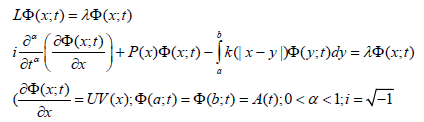(1)

The function P(x) is a continuous function, while k (|x-y)| is a discontinuous function. U is a constant and is a parameter

The equations of the form Lφ=λφ arise in many mathematical physics problems. It is often true that, the special solutions called EFs or characteristic functions. These EFs must not identically zero and satisfy one or more conditions that are supplementary related to the problem being solved. The EFs exist only for special values of the parameter λ; these values of λ are called EVs or characteristic values.

Consider Φm is a solution of the equation LΦmmΦm, λm is an eigenvalue, which is not identically zero and which satisfies the supplementary conditions, then Φm is called an EF belonging to the EVλm. Here, in this paper, the asymptotic behavior of the EVs and EFs for the linear operator will be discussed. In addition, some results will be considered. In section 2 the basic concepts of the linear integral operator is considered. Moreover, the Riemann – Liouville, and the Caputo derivatives of order fractional integral are considered. In section 3, using the separation variable method and the linear differential method, we obtain the general solution of the nuclear integro differential equation. Some important results for EVs and EFs are considered.

#### Basic Concepts

Definition (1): The integral operator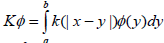where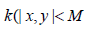or satisfies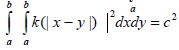in L2[a,b], is bounded and continuous. Moreover, the integral operator is called compact.

Lemma 1: The kernel k (x− y ),a ≤ x, y ≤ b, can be decomposed in an infinite number of ways, into the sum of the suitable degenerate kernel k0 (x , y) and another continuous kernel k1 (x , y) whose norm ||k1|| can be made small as we wish i.e.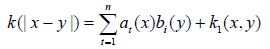Where ai(x), bi (y) and k1(x, y) are uniformly continuous and hence bounded in the interval [a,b].

Theorem1: Let H be L2(a,b) and K a degenerate integral operator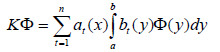K is a compact operator, if ai(x) and bi(x) belong to L2(a,b) for all i.

Theorem 2: Let (Kn) be a sequence of compact operators on a Hilbert space H, such that for some operator K we have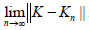. Then, K is also compact i.e.,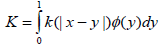is a compact operator on L2[0,1].

Definition (2): The Riemann -- Liouville fractional integral of order α>0, of the function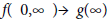is given by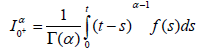Provided that the R.H.S is point wise definition (0,∞)

Definition (3): The Caputo derivatives of order α>0 of a continuous function f:(0,∞)→ g(∞) is given by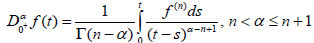Theorem 3: Let K be a compact operator on the Hilbert space H, and let {ψn} be a linearly independent sequence of EVs corresponding to some nonzero eigenvalue μ, that is Kψn=μψn for all n. Then, {ψn} contains a finite number of elements.

#### Method of Solution

Consider the general solution of (1) in the form: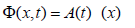(2)

Then, using (2) in (1), with aid of the boundary conditions, we have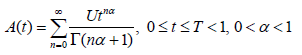(3)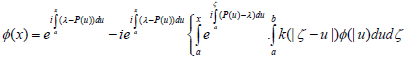(4)

Hence, the general solution of (1) is given as: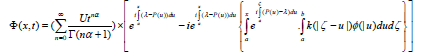(5)

The formula (5) represents a unique solution of the integro differential equation. To prove the boundedness and the orthogonally of the integro differential operator, we must know some properties of the fractional integro differential equations and the famous properties of the IE. Therefore, we consider eqn. (1) where p(x) is a real continuous function in (a,b), the kernel k (x − y) is continuous in the same interval. Moreover we have i.e.,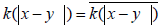and λ is real.

Definition (4): The kernel (t −τ )α −1,∀t,τ ∈[0,T],T <1,0 <α <1 satisfies for every continuous function h(τ) and all 1 2 0 ≤τ ≤τ ≤ t the integrals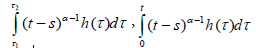are continuous functions of t, i.e.,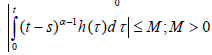is bounded.

Lemma 2: For the integro differential operator L of (1) and for every λ, the EFs of L, is bounded under the conditions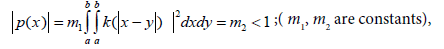Proof: Taking the norm of both sides of (2)-(4) then using the two conditions of p(x) and k(x,y) with the famous relation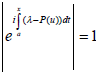, we have

Hence, we have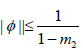and after using definition (3), we have ||A|| ≤ M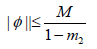(6)

The formula (6) proves the boundedness of the function Φ(x,λ;t) for all values of λ and x ∈[a,b],t ∈[0,T ],T <1.

Lemma 3: The EFs of the operator (1) corresponding to distinct EVs are orthogonal.

Proof: Assume that Φ1 (x; t) and Φ2 (x; t) are two real EFs corresponding to the two different eigenvalues λ1 and λ2 respectively i.e., we have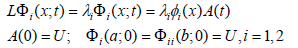(7)

Integrating (1) with respect to t, we get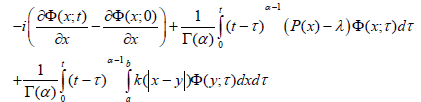(8)

Assuming that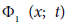and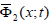satisfy eqn. (8). Then, multiplying the result by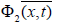and by Φ1 (x; t). Then subtracting the results and integrating with respect to x from a to b, then using the symmetric of the kernel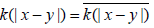Finally, we obtain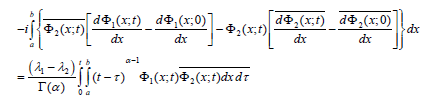(9)

After integrating and using the boundary conditions, the term in the left hand side will vanish. Hence, we get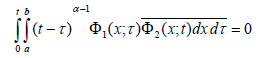(10)

The formula 10) represents the condition of the orthogonally of the EFs in the space [L2 [a,b] XC (0,T ),T<1. Moreover, the function of time (t−τ ) ,0 <α<1 is called the weight function.

Lemma 4: The EVs of the integro-differential operator (1) are real

Proof: Write the integro-differential operator L in the form of two operators, one is differentiable and the second is integrable i.e.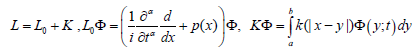Since L0 is self adjoint operator with the condition Φ(a;t)=Φ(b;t), see Abdou . In addition, the kernel of the second integral operator is symmetric then K is self adjoint. In many mathematical physics investigation and computation of a function f (λ) the neighborhood of a finite point λ0 or in the neighborhood of the point at infinity is connected with considerable difficulties, the expression of the neighborhood of the point at infinity means for λ→∞ or λ→∞. These difficulties may often be overcome by means of an asymptotic formula that substitutes a simpler function for the given function f (λ). This simpler function is chosen in such a manner that it can be investigated and computed in an easier way than the original function f (λ). Moreover, it approximates to an arbitrary degree of accuracy when λ tends to λ0 or approaches infinity.

Now, let we have the interval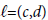and consider the functions f (λ), g(λ), h(λ), m(λ),... , defined on the interval (c,d). So we can define the following:

(1) If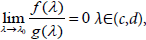we say that f (λ) is of higher orders of smallness than g(λ) on the interval (c,d) for λ→λ0 and write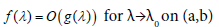(12)

(2) if there is a constant β, 0<β<∞, such that for all λ∈(c,d) belonging to a sufficiently small neighborhood of λ0, the inequality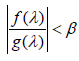holds, we say that, f (λ) is of the order of g(λ)on the interval (c,d) and write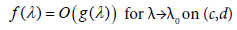(13)

(3) If the inequality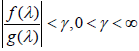is full-filled for all λ∈(c,d), we say that f (λ) is of the order of g(λ)

(4) We say that the two functions f (λ) and g(λ) are equivalent for λ→λ0 on the interval (c,d) and write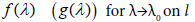(14)

The relation (14) can be adapted in the form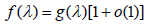(15)

The formula (15) is called an asymptotic representation of f (λ) in the neighborhood of the point λ0 on the interval (c,d). The reader must know that the asymptotically equivalent functions on unbounded interval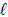in the neighborhood of the point at infinity, i.e., for λ→∞, is defined similarly.

Theorem 4: Let g(x) be an integrable function in the interval [γ,β] and μ be a parameter, then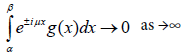Theorem 5: For the boundary value problem of (1), the EVs and EFs are asymptotically equivalent on the interval [a, b] to the EVs and EFs of the boundary value problem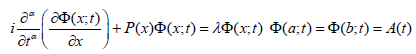(16)

Proof: Write the solution of (5), after using (2) and the condition Φ(a;t)=1 in eqn. (5) we have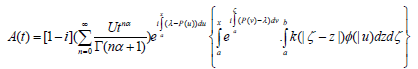(17)

Using the notations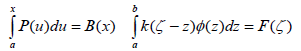(18)

then, using the second condition, Φ(b;t)=A(t) , in eqn. (5) we get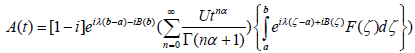(19)

The second term in the right hand side (19) consists of the functional ei{λ(b−a)−B(b)} which is bounded in the interval (a,b), also the function eiB(ζ) F(ζ),ζ∈(a,b) is an integrable function in (a,b). Then, by theorem (5), as λ→∞, the second term of (17) tends to zero. Thus for large value of λ, the formula (17) becomes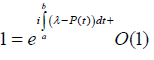(20)

Therefore, for large value of λ the roots of (20) becomes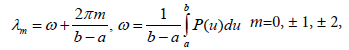(21)

This can be adapted in the form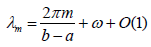(22)

The corresponding EFs are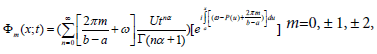The two formulas (22) and (23) represent, respectively the EVs and EFs of (1), and the general solution is given by: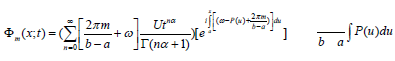(24)

#### Conclusion

Figure 1 represents the 3D-plot for the EFsΦ with different values α and m. For fixed values of x=0.5, the increasing of fractional parameter α∈(0,1) implies to increase the profile of the EFs. Figure 2 represents the 3D-plot for the EFs Φ with different values α and x. For fixed values of m, the increasing of fractional parameter α∈(0,1) implies to increase the profile of the EFs, the peak of the vibration waves at large values of α be greater than others. Figures 3 and 4 represent the 3D-plot for the EFs Φ with different values α and x at (m=10000, m=0) respectively. For fixed values of, by comparing the result in Figure 2 with the result in Figure 3, the effect of fractional parameter α∈(0,1) has the same effect on the EFs and the peak of the vibration wave. Figures 5-8 represent the 3D plot between (Φ, m, x) at different values α=1.0, 0.9,0.5,0.1 of on respectively. The effect of the fractional order parameter is very much prominent.

#### Future Work

In the future work the solution of nuclear integral equation in the homogeneous case, will be discussed and proved.

#### References

Select your language of interest to view the total content in your interested language

### Article Usage

• Total views: 1309
• [From(publication date):
June-2017 - Nov 17, 2019]
• Breakdown by view type
• HTML page views : 1184Can't read the image? click here to refresh